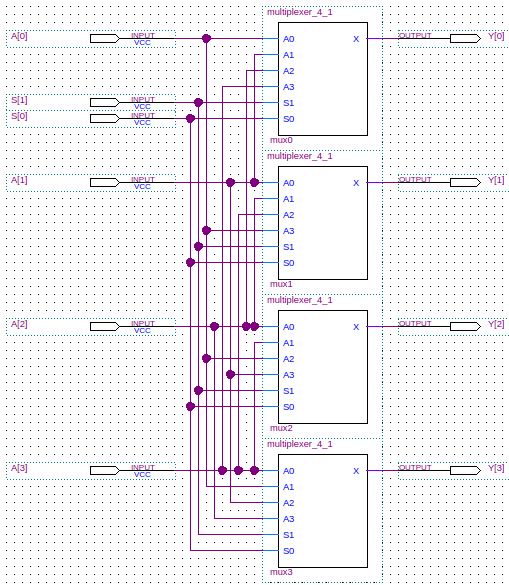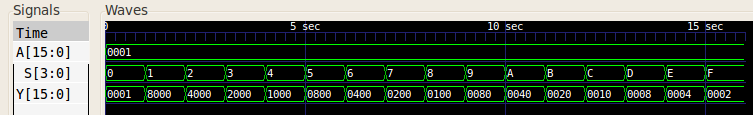# Shifter (barrel)

A barrel shifter is a combinational logic circuit which shifts the bits of a value in a given direction. The barrel shifter's function is similar to that of the shifter and the bidirectional shift register. However, the shifter only shifts one place in either direction whereas the barrel shifter can shift many places, and the register is a sequential logic circuit and this makes it unsuitable to be used in a function unit.

The basic n-bit barrel shifter design comprises n n-to-1 multiplexers, one multiplexer per input bit. Each multiplexer has each bit of the shifter's inputs as its inputs. To which multiplexer lines the shifter's input bits are connected determines the direction of the shift. Take for example an n-bit barrel shifter which has an n-bit input `A`, and shifts right. If we consider bit m, then multiplexer m's input line 0 would be connected to `A[m]`. Input line x would be connected to `A[(m+x) mod n]`. The table below shows how the multiplexers would be configured for a 4-bit barrel shifter which shifted right:

Multiplexer Input Line
Multiplexer number `0``1``2``3`
`0` `A``A``A``A`
`1` `A``A``A``A`
`2` `A``A``A``A`
`3` `A``A``A``A`

Because we use the mod operation, we see that the the least significant bit which is shifted out, is shifted back in again as the most significant bit — we essentially rotate the bits of the input. The table below shows how the multiplexer selection input (`S`) determines how many bits we shift:

`S`Bits shifted
`00`0 (No shift)
`01`1
`10`2
`11`3

From the description above we see that the barrel shifter shifts the bits in only one direction. However, a shift in the other direction by x places can be acomplished by a shift of n-x places. So, if we have a 4-bit barrel shifter that shifts right, we can shift left by one place by shifting right three places (3 = 4-1).

## Example

The schematic for a barrel shifter is shown below. To save space, a 4-bit barrel shifter design is shown. Here the shifted output is the 4-bit value `Y`. The 2-bit input value `S` is the multiplexer selector.## Verilog

Below is the Verilog code for a structural model of a 16-bit barrel shifter. The code is also shown for the multiplexer.

```	```
module barrel_shifter_16(Y, A, S);
output [15:0] Y;  // The shifted result.
input [15:0]  A;  // The value to be shifted.
input [3:0] 	 S;  // The amount to shift.

multiplexer_16_4 #(1) mux0(Y,
A, A, A, A, A, A, A, A,
A, A, A, A, A, A, A, A,
S, S, S, S);
multiplexer_16_4 #(1) mux1(Y,
A, A, A, A, A, A, A, A,
A, A, A, A, A, A, A, A,
S, S, S, S);
multiplexer_16_4 #(1) mux2(Y,
A, A, A, A, A, A, A, A,
A, A, A, A, A, A, A, A,
S, S, S, S);
multiplexer_16_4 #(1) mux3(Y,
A, A, A, A, A, A, A, A,
A, A, A, A, A, A, A, A,
S, S, S, S);
multiplexer_16_4 #(1) mux4(Y,
A, A, A, A, A, A, A, A,
A, A, A, A, A, A, A, A,
S, S, S, S);
multiplexer_16_4 #(1) mux5(Y,
A, A, A, A, A, A, A, A,
A, A, A, A, A, A, A, A,
S, S, S, S);
multiplexer_16_4 #(1) mux6(Y,
A, A, A, A, A, A, A, A,
A, A, A, A, A, A, A, A,
S, S, S, S);
multiplexer_16_4 #(1) mux7(Y,
A, A, A, A, A, A, A, A,
A, A, A, A, A, A, A, A,
S, S, S, S);
multiplexer_16_4 #(1) mux8(Y,
A, A, A, A, A, A, A, A,
A, A, A, A, A, A, A, A,
S, S, S, S);
multiplexer_16_4 #(1) mux9(Y,
A, A, A, A, A, A, A, A,
A, A, A, A, A, A, A, A,
S, S, S, S);
multiplexer_16_4 #(1) mux10(Y,
A, A, A, A, A, A, A, A,
A, A, A, A, A, A, A, A,
S, S, S, S);
multiplexer_16_4 #(1) mux11(Y,
A, A, A, A, A, A, A, A,
A, A, A, A, A, A, A, A,
S, S, S, S);
multiplexer_16_4 #(1) mux12(Y,
A, A, A, A, A, A, A, A,
A, A, A, A, A, A, A, A,
S, S, S, S);
multiplexer_16_4 #(1) mux13(Y,
A, A, A, A, A, A, A, A,
A, A, A, A, A, A, A, A,
S, S, S, S);
multiplexer_16_4 #(1) mux14(Y,
A, A, A, A, A, A, A, A,
A, A, A, A, A, A, A, A,
S, S, S, S);
multiplexer_16_4 #(1) mux15(Y,
A, A, A, A, A, A, A, A,
A, A, A, A, A, A, A, A,
S, S, S, S);
endmodule // barrel_shifter_16

module multiplexer_16_4(X, A0, A1, A2, A3, A4, A5, A6, A7, A8, A9, A10, A11, A12, A13, A14, A15, S3, S2, S1, S0);
parameter WIDTH=16;     // How many bits wide are the lines

output [WIDTH-1:0] X;   // The output line

input [WIDTH-1:0]  A15;  // Input line with id 4'b1111
input [WIDTH-1:0]  A14;  // Input line with id 4'b1110
input [WIDTH-1:0]  A13;  // Input line with id 4'b1101
input [WIDTH-1:0]  A12;  // Input line with id 4'b1100
input [WIDTH-1:0]  A11;  // Input line with id 4'b1011
input [WIDTH-1:0]  A10;  // Input line with id 4'b1010
input [WIDTH-1:0]  A9;   // Input line with id 4'b1001
input [WIDTH-1:0]  A8;   // Input line with id 4'b1000
input [WIDTH-1:0]  A7;   // Input line with id 4'b0111
input [WIDTH-1:0]  A6;   // Input line with id 4'b0110
input [WIDTH-1:0]  A5;   // Input line with id 4'b0101
input [WIDTH-1:0]  A4;   // Input line with id 4'b0100
input [WIDTH-1:0]  A3;   // Input line with id 4'b0011
input [WIDTH-1:0]  A2;   // Input line with id 4'b0010
input [WIDTH-1:0]  A1;   // Input line with id 4'b0001
input [WIDTH-1:0]  A0;   // Input line with id 4'b0000
input 	      S3;   // Most significant selection bit
input 	      S2;
input 	      S1;
input 	      S0;   // Least significant selection bit

assign X = (S3 == 0
? (S2 == 0
? (S1 == 0
? (S0 == 0
? A0       // {S3,S2,S1,S0} = 4'b0000
: A1)      // {S3,S2,S1,S0} = 4'b0001
: (S0 == 0
? A2       // {S3,S2,S1,S0} = 4'b0010
: A3))     // {S3,S2,S1,S0} = 4'b0011
: (S1 == 0
? (S0 == 0
? A4       // {S3,S2,S1,S0} = 4'b0100
: A5)      // {S3,S2,S1,S0} = 4'b0101
: (S0 == 0
? A6       // {S3,S2,S1,S0} = 4'b0110
: A7)))    // {S3,S2,S1,S0} = 4'b0111
: (S2 == 0
? (S1 == 0
? (S0 == 0
? A8       // {S3,S2,S1,S0} = 4'b1000
: A9)      // {S3,S2,S1,S0} = 4'b1001
: (S0 == 0
? A10      // {S3,S2,S1,S0} = 4'b1010
: A11))    // {S3,S2,S1,S0} = 4'b1011
: (S1 == 0
? (S0 == 0
? A12      // {S3,S2,S1,S0} = 4'b1100
: A13)     // {S3,S2,S1,S0} = 4'b1101
: (S0 == 0
? A14      // {S3,S2,S1,S0} = 4'b1110
: A15)))); // {S3,S2,S1,S0} = 4'b1111
endmodule // multiplexer_16_4
```
```

When given test inputs, the 16-bit barrel shifter generated the following waveform:## References

Mano, M. Morris, and Kime, Charles R. Logic and Computer Design Fundamentals. 2nd Edition. Prentice Hall, 2000.